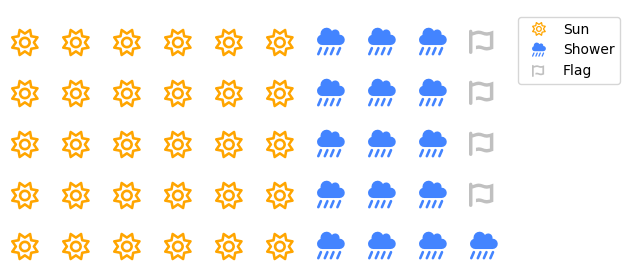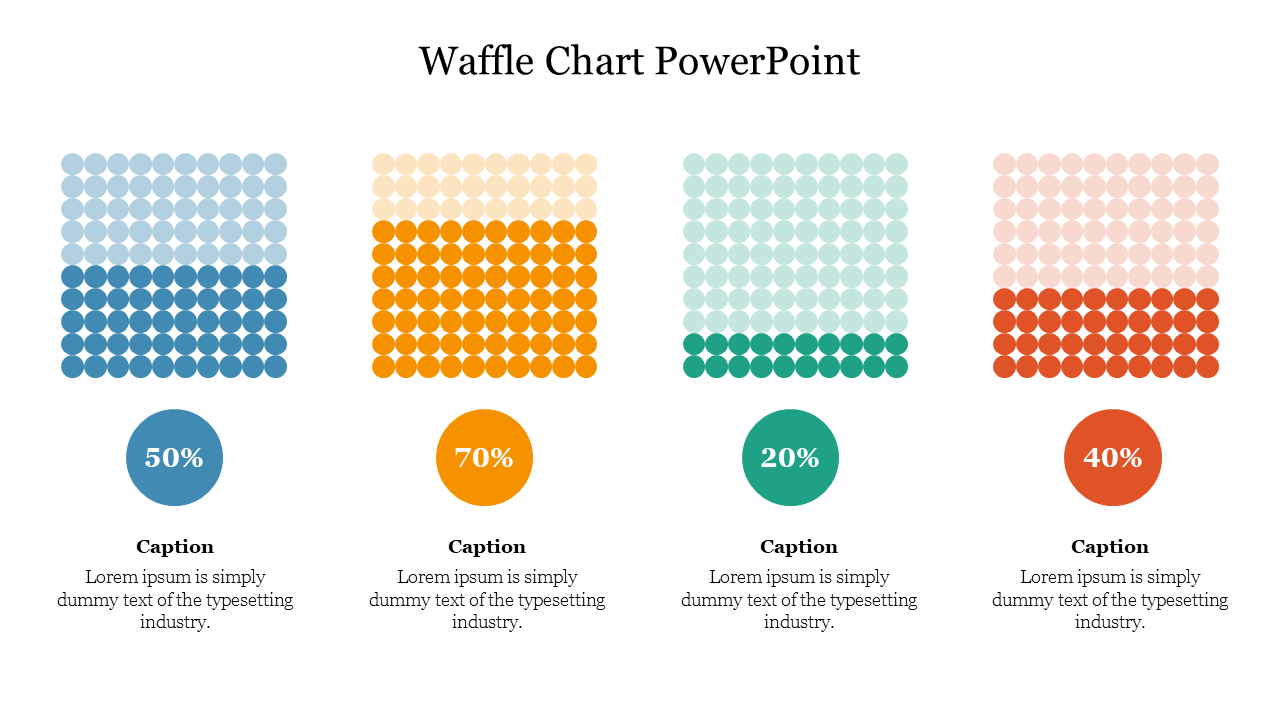1. 显示占比关系： 华夫图适用于呈现不同类别之间的占比关系。通过将矩形分割成小块，每个小块的大小与类别的相对占比成比例，观察者可以一目了然地看到各个类别的相对大小。
2. 易于理解： 华夫图的可视化形式非常直观，不需要复杂的解释即可传达数据信息。这使得它在简单地传达比例信息时非常有用。
3. 可用于分类和分组： 华夫图可用于显示多个类别或分组的占比关系。它适用于呈现类别的分布情况，例如不同地区的销售份额、产品类别的销售比例等。
4. 美观性： 华夫图的可视化效果通常很吸引人，能够吸引观众的注意力，从而更好地传达信息。
5. 简化复杂数据： 华夫图可以用于将复杂的数据转化为简单的形式，以便更好地展示主要的占比关系，而不必深入细节。
6. 与颜色编码相关性： 华夫图可以通过为每个小块选择不同的颜色来传达额外的信息。这可以用于显示不同类别之间的相关性、区分特定的子类别等。

PyWaffle是一个开源的、使用MIT许可的Python包，用于绘制华夫饼图。 提供了一个名为"Figure"的构造类"Waffle"，可以传递给matplotlib.pyplot.figure，并生成一个matplotlib的"Figure"对象。

## 安装#

!pip3 install pywaffle


## 一、快速入门#

### 1.1 列表数据#

import matplotlib.pyplot as plt
from pywaffle import Waffle

fig = plt.figure(
FigureClass = Waffle,
rows = 5,
columns = 10,
values = [30, 16, 4]
)

fig.savefig('plot1.png', dpi=300)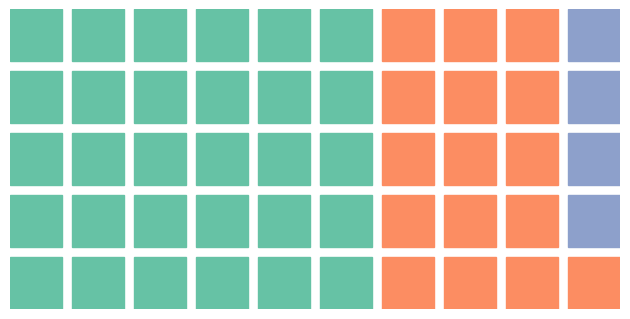### 1.2 字典数据#

fig = plt.figure(
FigureClass = Waffle,
rows = 5,
columns = 10,
values = {'Cat1': 30,
'Cat2': 16,
'Cat3': 4},
legend = {'loc': 'upper left', 'bbox_to_anchor': (1, 1)}
)

fig.savefig('plot2.png', dpi=300)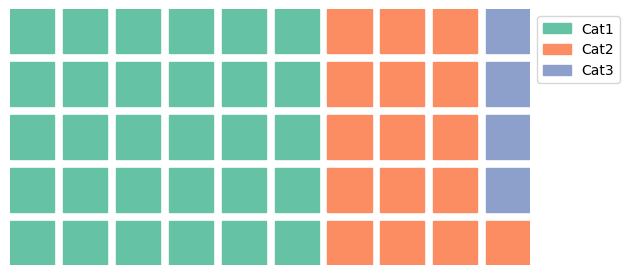### 1.3 DataFrame数据#

import pandas as pd

data = [30, 16, 4]
df = pd.DataFrame(data,
columns=['Value'],
index=['Cat1', 'Cat2', 'Cat3'])

fig = plt.figure(
FigureClass=Waffle,
rows=5,
columns=10,
values=df['Value'],
labels=list(df.index),  # 没有这行， Legend 不会显示
legend={'loc': 'upper left', 'bbox_to_anchor': (1, 1)}
)fig = plt.figure()

# 修改已存在的axis
ax.set_title("Axis Title")
# 确保绘制的图形在坐标轴两个方向上的比例保持一致
ax.set_aspect(aspect="equal")

Waffle.make_waffle(
ax=ax,  # pass axis to make_waffle
rows=5,
columns=10,
values=[30, 16, 4],
title={"label": "Waffle Title", "loc": "left"}
)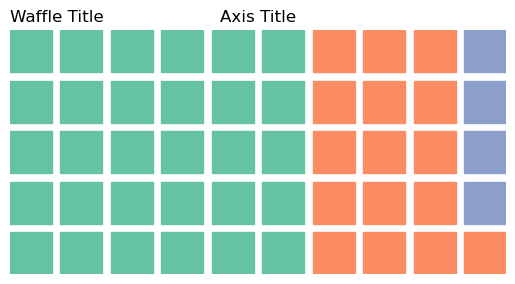## 二、值缩放&尺寸调整#

### 2.1 数值缩放#

fig = plt.figure(
FigureClass=Waffle,
rows=5,
columns=10,
values=[48, 46, 3],
rounding_rule='floor'
)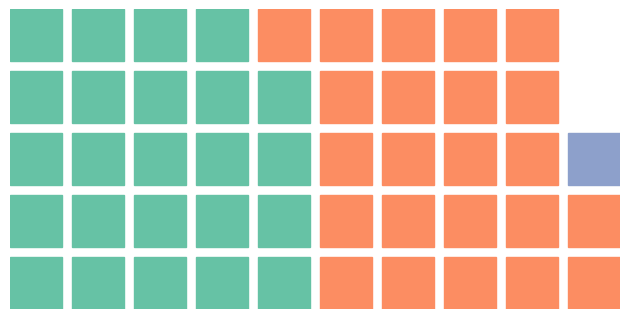### 2.2 自动调整尺寸#

fig = plt.figure(
FigureClass=Waffle,
rows=5,
values=[48, 46, 3]
)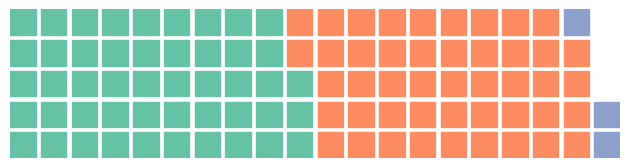## 三、标题、标签、图例#

• 参数 title 以字典形式接受 matplotlib.pyplot.title 的参数。
• 参数 labels 以列表中的字符串标签形式接受。如果未指定，将使用 values 的键作为标签。
• 参数 legend 以字典形式接受 matplotlib.pyplot.legend 的参数。

data = {'Cat1': 30, 'Cat2': 16, 'Cat3': 4}

fig = plt.figure(
FigureClass=Waffle,
rows=5,
columns=10,
values=data,
title={
'label': 'Example plot',
'loc': 'center',
'fontdict': {
'fontsize': 20
}
},
labels=[f"{k} ({int(v / sum(data.values()) * 100)}%)" for k, v in data.items()],
legend={
# 'labels': [f"{k} ({v}%)" for k, v in data.items()],  # lebels could also be under legend instead
'loc': 'lower left',
'bbox_to_anchor': (0, -0.4),
'ncol': len(data),
'framealpha': 0,
'fontsize': 12
}
)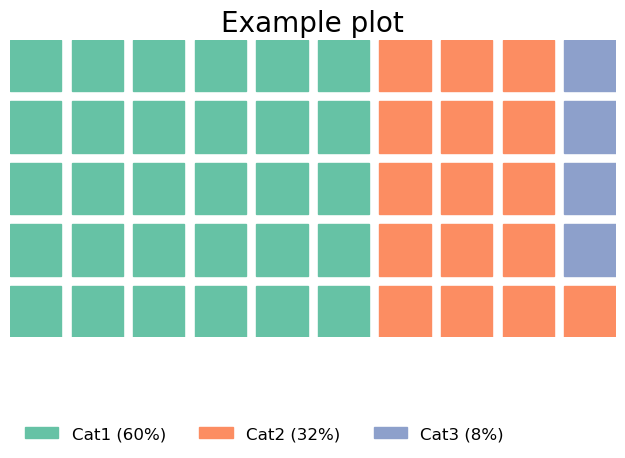### 四、块配色#

fig = plt.figure(
FigureClass=Waffle,
rows=5,
columns=10,
values=[30, 16, 4],
colors=["#232066", "#983D3D", "#DCB732"]
)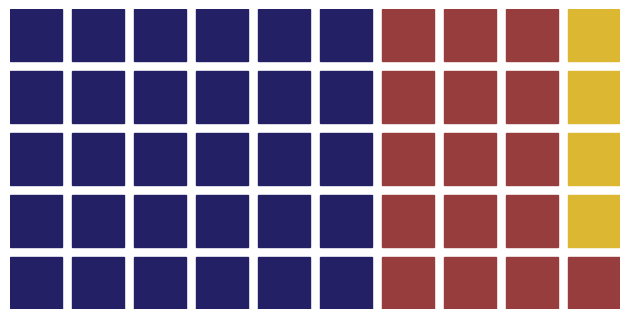fig = plt.figure(
FigureClass=Waffle,
rows=5,
columns=10,
values=[30, 16, 4],
cmap_name="tab10"
)## 五、块形状#

### 5.1 characters#

fig = plt.figure(
FigureClass=Waffle,
rows=5,
values=[30, 16, 4],
colors=["#4C8CB5", "#B7CBD7", "#C0C0C0"],
characters='❤',
font_size=24
)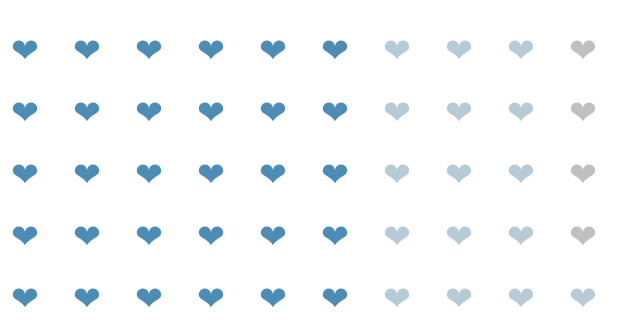### 5.2 图标#

PyWaffle 支持通过 Font Awesome 绘制带有图标的图表。有关 Font Awesome 如何集成到 PyWaffle 中的信息，请查看“Font Awesome 集成”页面。要在 Font Awesome 中搜索可用的图标名称，请访问 https://fontawesome.com/search

fig = plt.figure(
FigureClass=Waffle,
rows=5,
values=[30, 16, 4],
colors=["#232066", "#983D3D", "#DCB732"],
icons='star',
font_size=24 #icon大小
)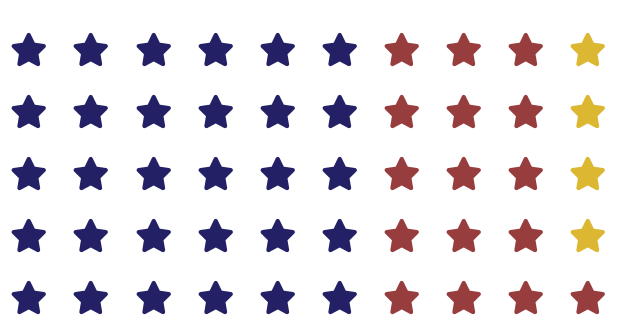fig = plt.figure(
FigureClass=Waffle,
rows=5,
values=[30, 16, 4],
colors=["#FFA500", "#4384FF", "#C0C0C0"],
icons=['sun', 'cloud-showers-heavy', 'snowflake'],
font_size=20,
icon_style='solid',
#设置 icon_legend=True，图例中的符号将会是图标。否则，它将是一个颜色条。
icon_legend=True,
legend={
'labels': ['Sun', 'Shower', 'Snow'],
'loc': 'upper left',
'bbox_to_anchor': (1, 1)
}
)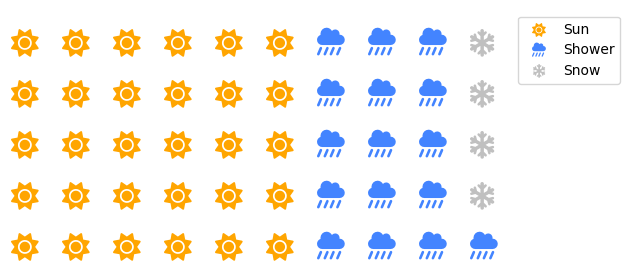Font Awesome根据图标名称和样式来定位图标。因此，不同图标的样式可能不同，您必须为所有图标单独指定图标样式。因此，icon_style 参数还可以接受一个包含样式字符串的列表或元组，以用于不同的图标。

fig = plt.figure(
FigureClass=Waffle,
rows=5,
values=[30, 16, 4],
colors=["#FFA500", "#4384FF", "#C0C0C0"],
icons=['sun', 'cloud-showers-heavy', 'font-awesome'],
icon_size=20,
icon_style=['regular', 'solid', 'brands'],
icon_legend=True,
legend={
'labels': ['Sun', 'Shower', 'Flag'],
'loc': 'upper left',
'bbox_to_anchor': (1, 1)
}
)